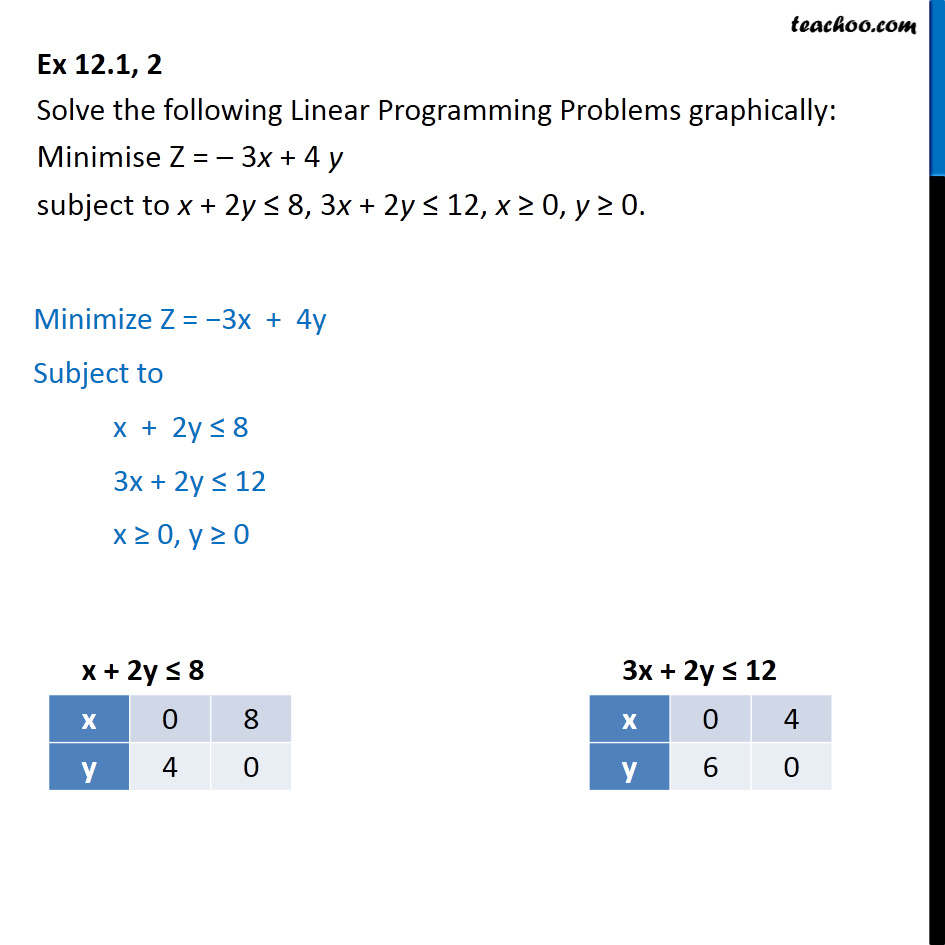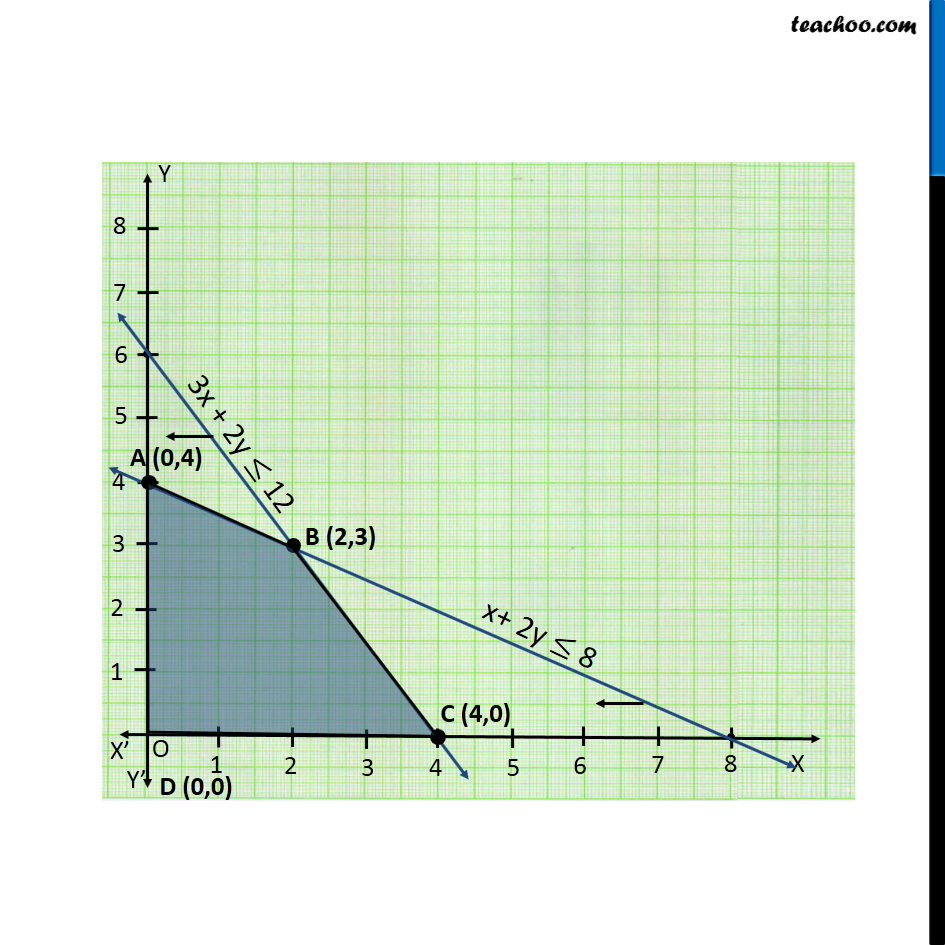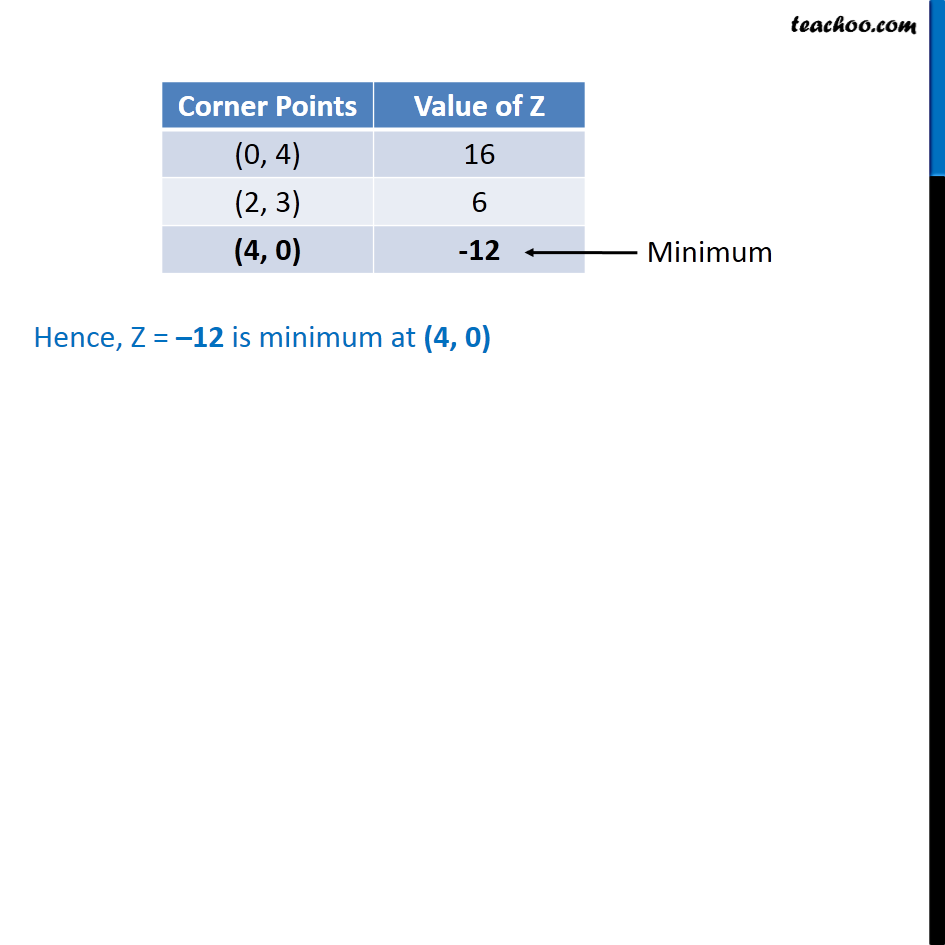Ex 12.1

Chapter 12 Class 12 Linear Programming
Serial order wiseLearn in your speed, with individual attention - Teachoo Maths 1-on-1 Class

### Transcript

Ex 12.1, 2 Solve the following Linear Programming Problems graphically: Minimise Z = – 3x + 4 y subject to x + 2y ≤ 8, 3x + 2y ≤ 12, x ≥ 0, y ≥ 0. Minimize Z = −3x + 4y Subject to x + 2y ≤ 8 3x + 2y ≤ 12 x ≥ 0, y ≥ 0 Hence, Z = –12 is minimum at (4, 0)